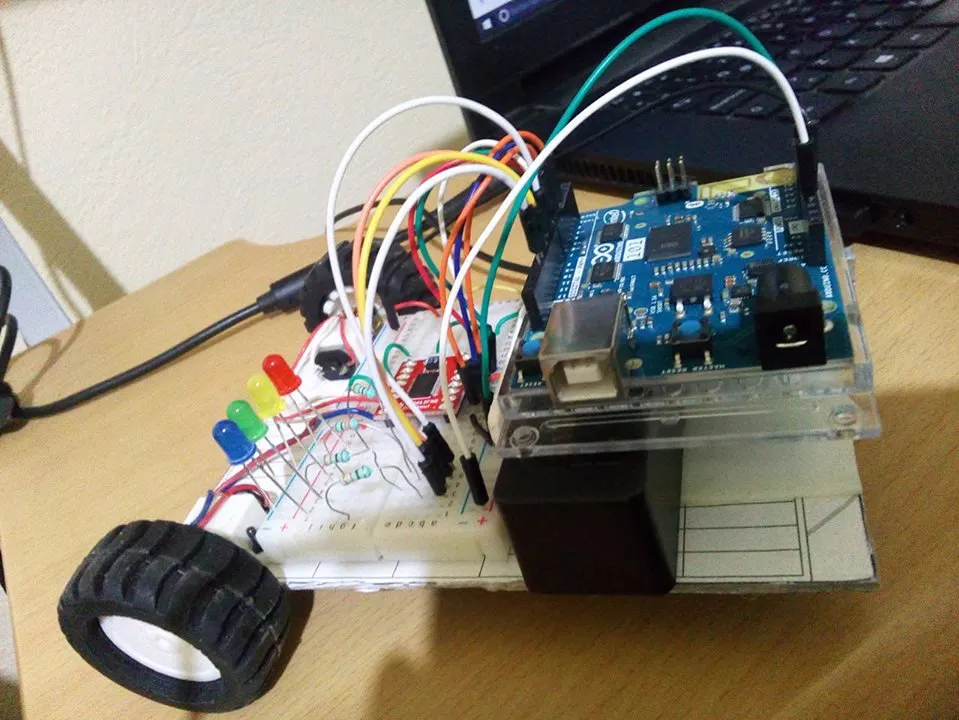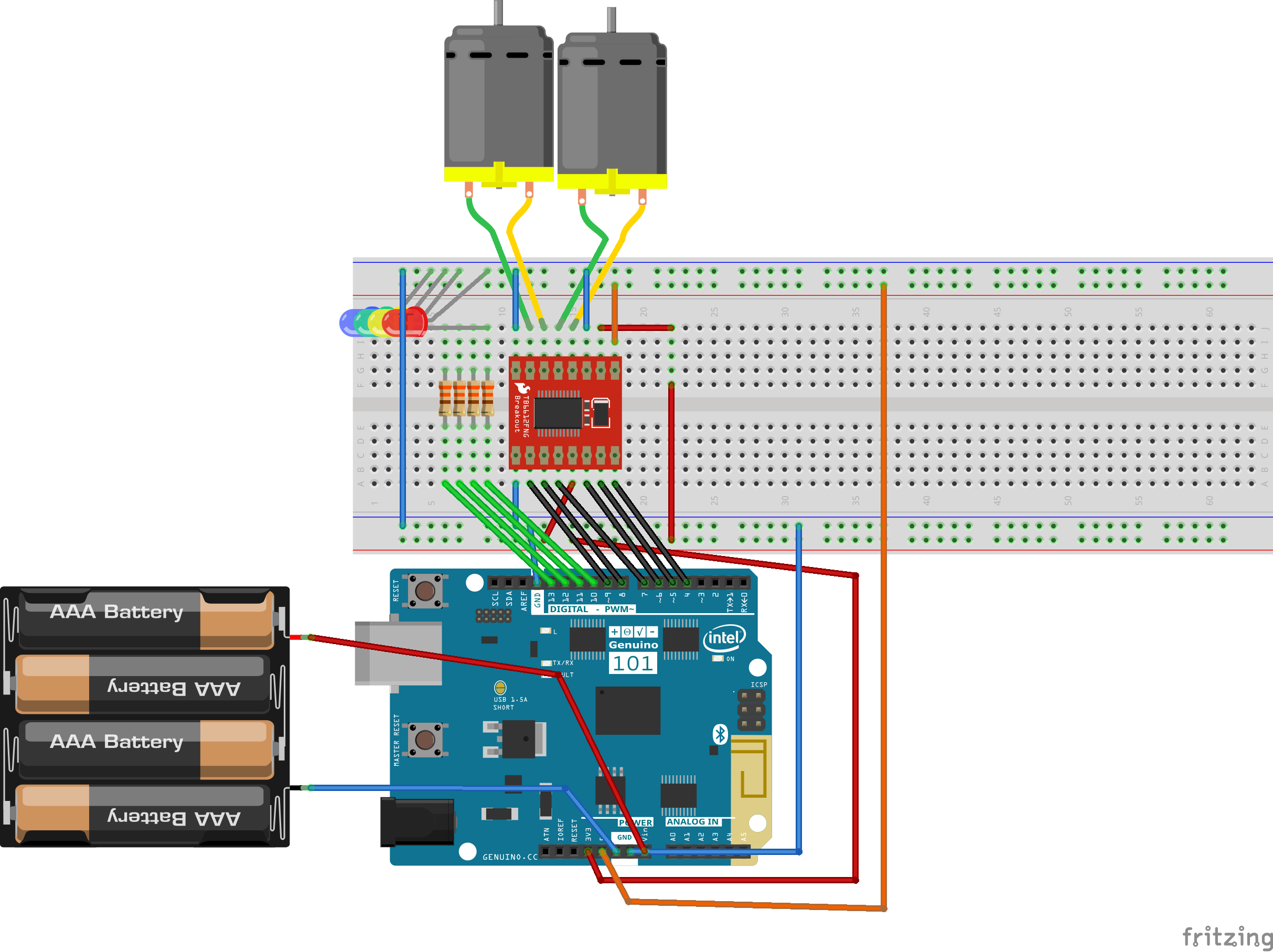Project tutorialMy First Arduino 101 Car Controlled by BLE © GPL3+

Recently I received an Arduino 101 kit from Hackster. My joy was great because it had a lot to do new projects!

• 3,923 views
• 14 respects

Components and supplies

Introduction

Some days ago I got my Arduino 101 kit that was given to me by Hackster and I decided to start doing projects and testing the new components that come in the kit. I decided to make a car controlled by Bluetooth and the Blynk application taking advantage of the Sparkfun motor driver.

I also used the Arduino 101 board that came included in the kit.

I use a pair of pololu engines with gearbox.

I also use an external smartphone battery that has a voltage of 5v and a current of 2600mAh that is enough for the entire circuit.

Result

The final design I liked a lot, I made it small and as I use the power bank instead of normal batteries like AA. I was able to save a lot of space and the weight of the final prototype was reduced. On the tires, use neoprene tires that stick very well to the ground and buy them in amazon. The third wheel is a crazy wheel that is super indispensable for the good movement of the car. The base of the cart is made of hard carton of an old notebook and a protoboard stuck in the carton, that's where the motor driver circuit is built. And I also put a few LEDs to make it look more colorful. These LEDs turn on and off randomly, the code is at the end if you want to check.

The control application of the car is done in Blynk and only added the Bluetooth function and added 4 buttons for the movement of the car (forward, reverse, clockwise rotation and counterclockwise), the application Is best handled in landscape view since ergonomics is lost in a vertical way.

Final

I am very happy with this project, I have now a new goal, to use this prototype and add functions like that of a line follower, and that of a robot dodge obstacles with ultrasonic sensors.

Code

Arduino car codeArduino
#define BLYNK_PRINT Serial
#include <BlynkSimpleCurieBLE.h>
#include <CurieBLE.h>

char auth[] = "5733e53afff240d79e49d7acc0849faa";

BLEPeripheral  blePeripheral;

const int DIR_A1 = 4;
const int DIR_B1 = 5;
const int PWM1 = 6;
const int DIR_A2 = 7;
const int DIR_B2 = 8;
const int PWM2 = 9;
const int luz = 13;
int pinValue1=0,pinValue2=0,pinValue3=0,pinValue4=0;
bool cambio=LOW;
byte random1;
const byte pin1=10,pin2=11,pin3=12,pin4=13;
long currentMillis;
long previousMillis = 0;

BLYNK_WRITE(V1)
{
pinValue1 = param.asInt(); // assigning incoming value from pin V1 to a variable

}
BLYNK_WRITE(V2)
{
pinValue2 = param.asInt(); // assigning incoming value from pin V1 to a variable

}
BLYNK_WRITE(V3)
{
pinValue3 = param.asInt(); // assigning incoming value from pin V1 to a variable

}
BLYNK_WRITE(V4)
{
pinValue4 = param.asInt(); // assigning incoming value from pin V1 to a variable

}

void setup()
{
// Debug console
Serial.begin(9600);
pinMode(DIR_A1, OUTPUT);
pinMode(DIR_B1, OUTPUT);
pinMode(PWM1, OUTPUT);
pinMode(DIR_A2, OUTPUT);
pinMode(DIR_B2, OUTPUT);
pinMode(PWM2, OUTPUT);
pinMode(luz, OUTPUT);
pinMode(pin1,OUTPUT);
pinMode(pin2,OUTPUT);
pinMode(pin3,OUTPUT);
pinMode(pin4,OUTPUT);
delay(1000);

blePeripheral.setLocalName("Blynk");
blePeripheral.setDeviceName("Blynk");
blePeripheral.setAppearance(384);

Blynk.begin(blePeripheral, auth);

blePeripheral.begin();

Serial.println("Waiting for connections...");

}

void loop()
{
blePeripheral.poll();
Blynk.run();
digitalWrite(luz, HIGH);
if(pinValue1==1)
{
digitalWrite(DIR_A1, LOW);
digitalWrite(DIR_B1, HIGH);
digitalWrite(DIR_A2, LOW);
digitalWrite(DIR_B2, HIGH);
analogWrite(PWM1, 255);
analogWrite(PWM2, 255);
}
else if(pinValue2==1)
{
cambio=HIGH;
digitalWrite(DIR_A1, HIGH);
digitalWrite(DIR_B1, LOW);
digitalWrite(DIR_A2, HIGH);
digitalWrite(DIR_B2, LOW);
analogWrite(PWM1, 255);
analogWrite(PWM2, 255);
}

else if(pinValue3==1)
{
cambio=HIGH;
digitalWrite(DIR_A1, HIGH);
digitalWrite(DIR_B1, LOW);
digitalWrite(DIR_A2, LOW);
digitalWrite(DIR_B2, HIGH);
analogWrite(PWM1, 100);
analogWrite(PWM2, 255);
}

else if(pinValue4==1)
{
cambio=HIGH;
digitalWrite(DIR_A1, LOW);
digitalWrite(DIR_B1, HIGH);
digitalWrite(DIR_A2, HIGH);
digitalWrite(DIR_B2, LOW);
analogWrite(PWM1, 255);
analogWrite(PWM2, 100);
}

else if(pinValue1==0 && pinValue2==0 && pinValue3==0 && pinValue4==0 )
{
digitalWrite(DIR_A1, HIGH);
digitalWrite(DIR_B1, LOW);
digitalWrite(DIR_A2, HIGH);
digitalWrite(DIR_B2, LOW);
analogWrite(PWM1, 0);
analogWrite(PWM2, 0);
}
random1 = random(10,14);
currentMillis = millis();
// if 10s have passed, check the sensor:
if (currentMillis - previousMillis >= 500) {
previousMillis = currentMillis;
digitalWrite(random1, estado) ;            // escribimos el nuevo valor
}

}

Schematics

Easy diagram• 89,343 views
• 135 respects

Arduino 101 BLE App

Project in progress by Alexis Santiago Allende

• 22,384 views
• 78 respects

Arduino 101 Home BLE System

Project tutorial by Alexis Santiago Allende

• 5,425 views
• 17 respects

• 4,887 views
• 6 respects

Bluetooth Controlled Car

Project tutorial by JANAK13

• 58,229 views